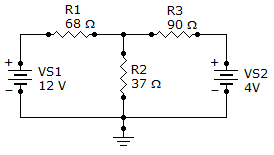# Electrical Engineering - Branch, Loop and Node Analyses - Discussion

Discussion Forum : Branch, Loop and Node Analyses - General Questions (Q.No. 6)
6.
What is the voltage drop across R1?850 mV
7.82 V
9.18 V
918 mV
Explanation:
No answer description is available. Let's discuss.
Discussion:
21 comments Page 1 of 3.

Dinkalem Mesfin said:   6 months ago
Applying KCL at node ------>(1)
i1-i2-i3=0
then in equation 1 apply KVL
12-Va/8 - Va/37 - (Va-4)/90 = 0.
50024 - 11966Va/ 226440 = 0.
Va=4.18

Then to find the voltage drop across R1.
Remember that voltage in sires is sum up.
Vt = V1+V2+V3+......Vn.
Va = 4.18,
Va = VR1 + (-12),
VR1 = 12-4.18,
VR1 = 7.82A.

Rajendra said:   4 years ago
Assume middle point as Va,
Calculate va
Then Vs1-Va = Voltage across R1.

Ron said:   6 years ago
Let VB the voltage drop across 68 resistor and let VA the node between 68 and 37.

Lets solve first the VA :
VA-12/68 + VA/37 + VA-4/90 =0.
VA = 4.18 V.
Using loop analysis :
12-VB-4.18 = 0.
VB = 7.819 V.

Chhatrapal said:   6 years ago
68/68 + 37 * 12 = 7.77 near to answer.

Lahu said:   6 years ago
First Calculate R1 current.
Using v = ir,
V = voltage drop across R1.

ALFRED said:   7 years ago
(VA - 12/68) + (VA - 4/90) + (VA/37) = 0.

=> (1.324 VA - 15.88/90) + (VA - 4/90) + 2.432 (VA/90) = 0

=> 1.324 VA + VA + 2.432 VA = 15.9 + 4

=> 4.75 VA = 19.9

=> VA = 19.9 / 4.75

=> 4.19#

=> 12 - 4.19 = 7.81#

Dhatrak Sonali said:   7 years ago
For loop 1.

= - 105I1 + 37I2 = - 12.....1st equation.

For 2nd loop.

37I1 - 127I2 = 4.

I1 = 0.114 A.

I2 = 0.002 A.

Voltage drop across R1.

V = R1*I1.

= 68*0.114.

V = 7.752 V.

BHEEMAN said:   7 years ago
Please solve this step by step with an equation.

Krishna said:   7 years ago
Using mesh analysis we get two equations we get the current passing through 1st loop as 0. 114 A which is the current flowing through R1.

So according to ohm's law V = IR thus the voltage drop across R1 is 0.114*68 the answer is 7.75 V.

Sysya said:   7 years ago
I don't get anything. Explain briefly.